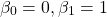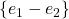# Volatility forecast evaluation in R

In portfolio management, risk management and derivative pricing, volatility plays an important role. So important in fact that you can find more volatility models than you can handle (Wikipedia link). What follows is to check how well each model performs, in and out of sample. Here are three simple things you can do:

1. Regression based test – Mincer Zarnowitz regression.
The idea is simple, regress actual (realized) values on forecasts:Now we jointly test the hypothesis:An intercept of zero means that your forecast in unbiased. Why? say by way of contradiction it is 0.02, this means that in order for the two sides to equate, we add to 0.02 on average to the forecast which means it is constantly under estimating the observed. The slope should be 1, as to say, your forecast “explains” the observed value perfectly.

2. Pairwise Comparison – Diebold Mariano test.
Say you have two models which produce two sets of forecasts. you therefore have two sets of errors. Call these errorsIn the case where the two methods are the same, the difference between these two vectorsis zero on average (Or a function of these vectors, e.g. e1^2 – e2^2). In the extreme case where you just replicate the forecasts using the same method, the difference is exactly zero. More importantly, we know how this difference is distributed (asymptotically..) so that we can test if it is indeed far from zero.

Students always have trouble understanding this point. The explanation I give is that it is impossible to measure the distance between 0 and 2 without knowing how likely a result of 2 really is! A result of 2 with a uniform distribution between {-3,3} is not as unlikely as a result of 2 with the standard normal distribution. I guess that is also the reason why we call the probability, a probability measure, since it measures..

The method that produces significantly smaller errors (typically squared errors or absolute errors) is preferred. You can easily extend it to more than one comparison (google “Tukey for all pairwise comparisons”, or look here for the multivariate extension of the Diebold Mariano test.)

3. Jarque–Bera test
In the case we have an accurate volatility forecast. We can center the series and scale it using our forecasts for the standard deviation. To be precise:Should have mean zero and standard deviation of one. The new standardized series will NOT be in general normally distributed, but you can use this test as a measure for how well the skewness and kurtosis of the original series are captured by your model. In fact, the idea for this post came to me when I read Pat’s post: garch-and-long-tails where Pat was checking how Kurtosis is (unconditionally) captured when we use t-distribution instead of normal in the Garch model. The Jarque–Bera test is a natural extension since the higher moments, skewness and kurtosis, appear in the expression for the test statistic.

In practice
The first two options are valid for general forecasting evaluation, however, volatility is unobservable so it is unclear what we use as observed values. What we do is to replace the “observed” with a proxy, typically the squared return. This proxy is known to be not very accurate. here you can find more accurate alternative, however, they are based on intra-day information so you need access to intra-day data source.

Let’s see how this works in R. Most of the code is written for us, which should remind you how nice it is to belong with the R community. We need:

I pull the data from google, make the return series for the S&P for the most recent 5 years and estimate the standard garch(1,1) and another more accurate GJR-garch (asymmetric garch) and implement the three options.

Notes
1. I did not really produce forecasts here but fitted values. For forecasting you need to create forecasts recursively using a loop for h step ahead forecast.

### 8 comments on “Volatility forecast evaluation in R”

1.ES says:

I tried to run your code in R after installing the requisite packages, and returned the following errors:

me.default(Tgjrmodel) :
cannot coerce class “structure(“uGARCHfit”, package = “rugarch”)” to a data.frame
> Nfit = as.data.frame(Nmodel)\$sigma
Error in as.data.frame.default(Nmodel) :
cannot coerce class “structure(“uGARCHfit”, package = “rugarch”)” to a data.frame
> ret.sq = ret^2
> N.sq = Nfit^2
> Tgjr.sq = Tgjrfit^2
> plot(ret.sq, ty = “l”, ylim = c(0,.004)) ; lines(Tgjr.sq, col = 2) ; lines(N.sq, col = 3)
>

What do you think the problem is?

I am trying to use the advice/examples you provided on a dataset that I have (dataframe with 1300 rows, 2 columns–data and security price) and I am having difficulties. Any help is appreciated.

1.Eran says:

Hi Eran,
First, I noticed there has been some changes in quantmod package so change:
ret[2:n] <- dat0[2:n,4]/dat0[1:(n-1),4] - 1 to: ret[2:n] <- as.numeric(dat0[2:n,4])/as.numeric(dat0[1:(n-1),4]) - 1 Second, I just re-did it, it works fine on my end. So my advice is to check R-version and make sure packages are updated. Good luck debugging.

1.ES says:

Thank you very much, Eran. I was about to e-mail you before I saw this response. I am going to ping you with some other questions if you don’t mind. Your blog is very insightful and helpful. Keep up the great work.

2.Fernando Aiube says:

Hi Eran,

I am reading your blog on testing out of sample performance of 2 different models.
I have some questions and I wondering if you could help me with them:
1) In the DM test in package forecast we can choose the function power 1 or 2.
Does this mean the MSE and the MAE loss functions using 2 and 1, respectively?
2) Is it common to find different reponses if we choose power function 1 or 2?
I mean, should the performance of the two models be the same regardless of the power function.
Fernando

1.Eran says:

Hi Fernando,

1) In the DM test in package forecast we can choose the function power 1 or 2.
Does this mean the MSE and the MAE loss functions using 2 and 1, respectively?

You can check it out yourself:
> print(dm.test)

d <- c(abs(e1))^power - c(abs(e2))^power .. So power is the exponent. What might be important for you is that the residual (e1 is the residual from model #1 and e2 is the residual from model #2), when it comes to volatility, can be confusing. It is the difference between the forecast of the volatility and the "observed" volatility. I write "observed" since we do not really see it as we see a price of a stock for example. What we see is a proxy for the unobserved volatility and we treat it as given. 2) Is it common to find different reponses if we choose power function 1 or 2? I mean, should the performance of the two models be the same regardless of the power function.

The exponent, may it be 1 or 2, reflects risk profile of the user. power=2 penalize the mistakes or the errors differently than power=1. Think about a deviation from the observed proxy of 10, in the power=2, penalty is 100 instead of 10. For values smaller than one the situation is reversed of course.

Hope this helps.

3.Long Vo says:

Hi Eran,
Could you be more specific on the interpretation of the tests’ results?
Specifically, are they consistent with each others?
Thanks,
Long

1.Eran Raviv says:

Hi Long,
Good question, thanks.

1. These tests do not have high power
2. They are not consistent with each other. Each captures different aspect.

4.yunusa says: